Author: Oscar Cronquist Article last updated on August 24, 2021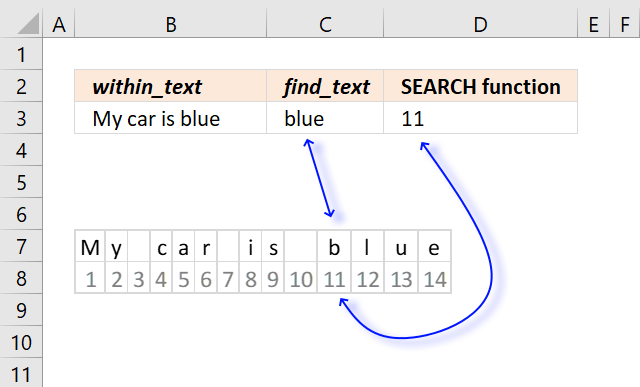The SEARCH function returns a number representing the position of character at which a specific text string is found reading left to right. It is not a case-sensitive search.

The image above demonstrates the function in cell D3.

=SEARCH(C3, B3)

The first argument find_text is a cell reference to the value in cell C3, the function will look for the value specified in cell C3 in the value in cell B3.

It returns number 11 in this example. Text string "blue" is found in position 11 if you count characters from left to right "My car is blue".

Row 7  shows the text string with a single character in each cell, row 8 shows the count for each cell.

## Excel function syntax

SEARCH(find_text,within_text, [start_num])

## Arguments

 find_text Required. Is the text you want to find. Wildcard characters (? and *) are allowed. within_text Required. Is the text in which you want to search for find_text. [start_num] Optional. Is the character number in within_text, counting from the left at which you want to start searching. If omitted 1 is used.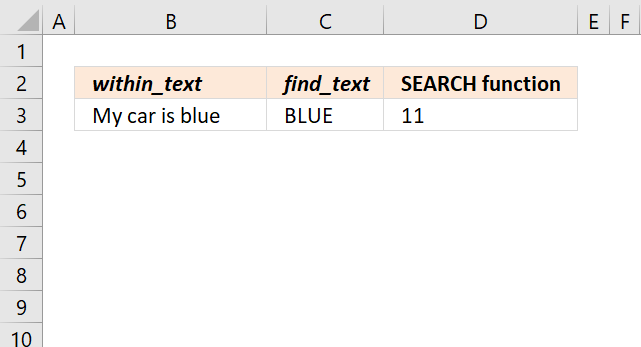The SEARCH function is not case sensitive, use the FIND function if lower and upper case letters are important.

The image above shows that using a text string in uppercase letters (cell C3) still matches the text in cell B3.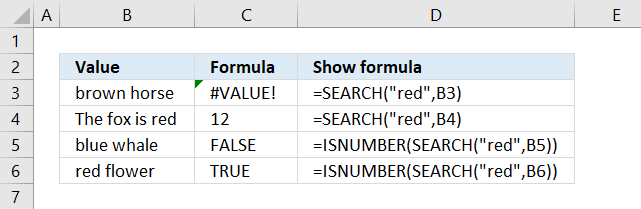Formula in cell C3:

=SEARCH("red", B3)

If the text string is not found the SEARCH function returns a #VALUE! error. The image above shows the erro output from the SEARCH function in cell C3.

Text string "red" is not found in cell B3 "brown horse", the issue with error values is that they break a formula, however, there are workarounds.

Use the ISNUMBER function to avoid breaking the formula. It returns TRUE if the returned value is a number and FALSE for everything else even errors.

Formula in cell C5:

=ISNUMBER(SEARCH("red", B5))

ISNUMBER(SEARCH("red", B5))

becomes

ISNUMBER(SEARCH("red", "blue whale"))

becomes

ISNUMBER(#VALUE!)

and returns FALSE in cell C5.

## 2. Wildcard characters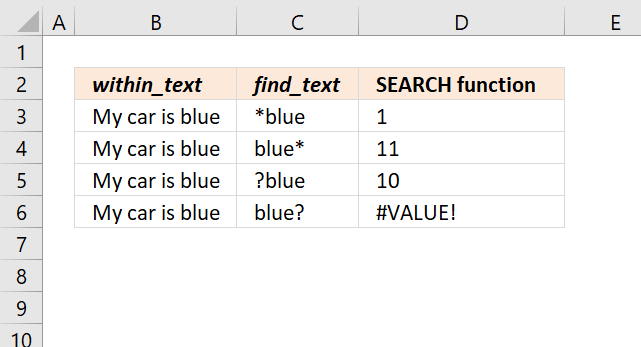There are two wildcard characters you can use in the SEARCH function.

 Character Wildcard Description * asterisk Matches 0 (zero) to any number of characters. ? question mark Matches one character only.

Formula in cell D3:

=SEARCH(C3, B3)

The SEARCH function in cell D3 returns 1, cell C3 contains *blue. The asterisk matches any number of characters even 0 (zero).

*blue matches the entire text string in cell B3 "My car is blue" and therefore matches the first character and returns 1.

Formula in cell D4:

=SEARCH(C4, B4)

Cell C4 contains blue* and matches at the eleventh character, the asterisk matches any number of characters from zero and up.

Formula in cell D5:

=SEARCH(C5, B5)

The question mark character matches any single character, ?blue matches "My car is blue" at the tenth character. The ? matches the blank (space) as well and the SEARCH function returns 10.

Formula in cell D6:

=SEARCH(C6, B6)

blue? doesn't match text "My car is blue" and returns #VALUE! error. The question mark matches any single character but string "My car is blue" has no character after blue.

## 3. Count how many times a specific string is found in a cell using the SEARCH function?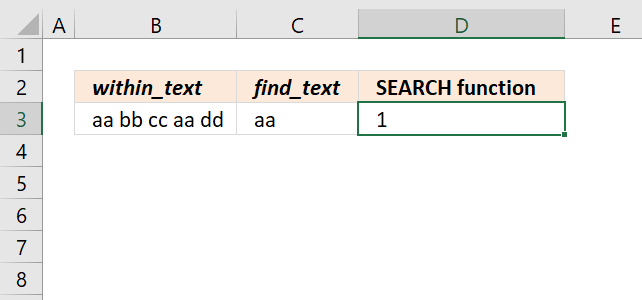You can't use the SEARCH function to count how many time a specific string exists in a cell, it will only return the number of the character of the string that first matches, reading from left to right.

This article explains how to Count specific text string in a cell.

## 4. Can you count how many times a specific string is found in a cell range using the SEARCH function?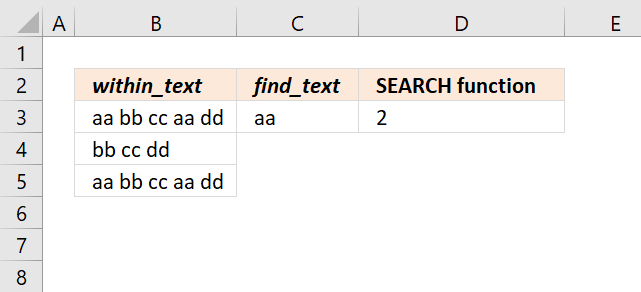Yes, but only one instance per cell. Formula in cell D3:

=SUM(ISNUMBER(SEARCH(C3,B3:B5))*1)

If you are an Excel 365 subscriber you can use the regular formula above. Excel users with previous versions can use the formula below.

=SUMPRODUCT(ISNUMBER(SEARCH(C3,B3:B5))*1)

Let me explain how these two formula work.

SEARCH(C3,B3:B5)

becomes

SEARCH("aa", {"aa bb cc aa dd"; "bb cc dd"; "aa bb cc aa dd"})

and returns {1; #VALUE!; 1}. The numbers indicate that a matching string is found.

ISNUMBER(SEARCH(C3,B3:B5))

becomes

ISNUMBER({1; #VALUE!; 1})

and returns {TRUE; FALSE; TRUE}. The SUM and SUMPRODUCT functions can't sum add boolean values, to convert boolean values to numbers simply multiply with 1.

ISNUMBER(SEARCH(C3,B3:B5))*1

becomes

{TRUE; FALSE; TRUE}*1

and returns {1;0;1}. 1 is the equivalent of boolean value TRUE and 0 (zero) is boolean value FALSE.

## 5. SEARCH function with multiple search values?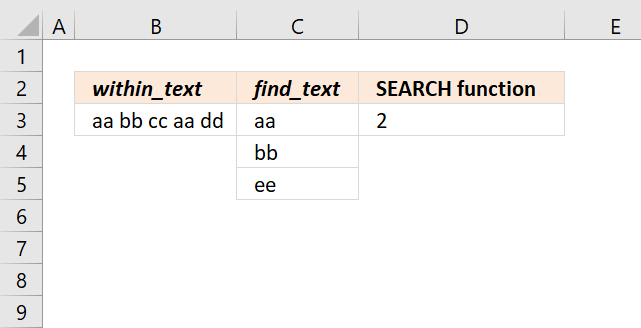You can use multiple text strings, however, if there are more than one instance of a specific string only one is counted.

For example cell B3 contains two "aa" but the formula in cell D3 counts only one instance.

=SUM(ISNUMBER(SEARCH(C3:C5, B3))*1)

The formula returns 2 because text strings "aa" and "bb" are found in cell B3. The formula is exactly the same as the one above so I won't explain it again, however, note that the cell references are different.

## 6. How to get the position of the second instance?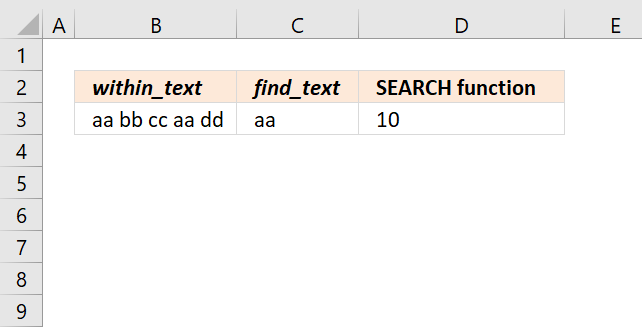The string "aa" we are looking for exists twice in cell B3, the following formula returns a number representing the position of the character of the string the second time it occurs.

=SEARCH(C3,REPLACE(B3,SEARCH(C3,B3),LEN(C3),REPT("|", LEN(C3))))

This formula changes the first instance to another string so that the SEARCH function then finds the position of the second instance of the string we are looking for.

SEARCH(C3,B3) finds the position of the first instance, it returns 1. The REPLACE function then changes the first string to something else, in this case, character "|". If your cell contains this character already then I recommend changing it to something that is not used in the cell so the formula doesn't get confused.

REPLACE(B3,SEARCH(C3,B3),LEN(C3),REPT("|", LEN(C3)))

becomes

REPLACE(B3,SEARCH(C3,B3),LEN(C3),REPT("|", LEN("aa")))

becomes

REPLACE(B3,SEARCH(C3,B3),LEN(C3),REPT("|", 2))

becomes

REPLACE(B3,SEARCH(C3,B3),LEN(C3),"||")

becomes

REPLACE(B3,SEARCH(C3,B3),2,"||")

becomes

REPLACE(B3,1,2,"||")

becomes

REPLACE("aa bb cc aa dd",1,2,"||")

and returns "|| bb cc aa dd".

SEARCH(C3,REPLACE(B3,SEARCH(C3,B3),LEN(C3),REPT("|", LEN(C3))))

becomes

SEARCH(C3,"|| bb cc aa dd")

and returns 10 in cell D3.

## 7. How to get the relative position of all instances?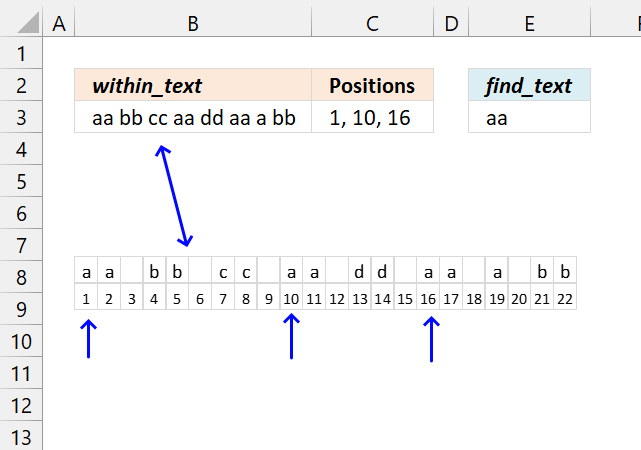The following formula works only in Excel 365, it returns numbers representing the position of the found instances in a cell.

Dynamic array formula in cell C3:

=TEXTJOIN(", ", TRUE, FILTER(SEQUENCE(LEN(B3)), MID(B3, SEQUENCE(LEN(B3)), LEN(E3))=E3))

### 7.1 Explaining formula in cell C3

LEN(B3)

#### Step 2 - Create a sequence from 1 to n

SEQUENCE(LEN(B3))

#### Step 3 - Create an array of substrings

MID(B3, SEQUENCE(LEN(B3)), LEN(E3))

#### Step 4 - Compare substrings to condition

MID(B3, SEQUENCE(LEN(B3)), LEN(E3))=E3

#### Step 5 - Filter corresponding numbers

FILTER(SEQUENCE(LEN(B3)), MID(B3, SEQUENCE(LEN(B3)), LEN(E3))=E3)

#### Step 6 - Concatenate strings

TEXTJOIN(", ", TRUE, FILTER(SEQUENCE(LEN(B3)), MID(B3, SEQUENCE(LEN(B3)), LEN(E3))=E3))

### Get the Excel fileSearch-and-Find-functions.xlsx